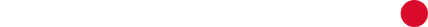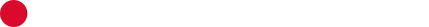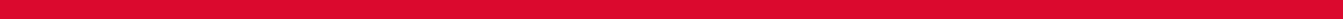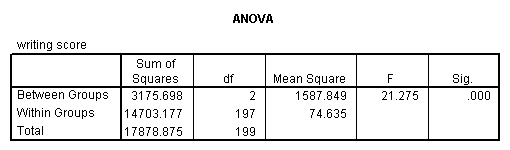### What is SPSS?

Statistical Product and Service Solutions (SPSS) is an advance statistical analysis software for students, researchers, Corporations and organizations. SPSS enables interactive statistical analysis encompassing descriptive and bivariate statistics. Coupled with inherent machine learning algorithms, it also enables data management and data documentation and is programmable through a 4GL command syntax language.### Key Components of SPSS#### SPSS Statistics

SPSS Statistics is an authoritative statistical software platform encouraging a valuable set of features enabling academia and organizations extract meaningful insights from data. SPSS Statistics is intended to resolve corporate and research problems using ad-hoc investigations and include advanced statistics, hypothesis testing, predictive analysis, regression, direct marketing, neural networks, complex samples, exact tests, decision trees, forecasting, and formulation of categories.#### SPSS Modeler

SPSS Modeler, the latest upgradation to the software’s fundamental framework, creates tighter integration with open source tools and easier model deployment of available resources. Organizations uses SPSS. Modeler to tap into data assets and modern applications, with complete algorithms and models that are ready for immediate use. SPSS Modeler has an additional feature of integration with verified IBM Watson Studio’s desktop, local, or Cloud.

• ##### Co-Variances To test the joint variability of 2 random variables#### One sample t-test

A one sample t-test allows us to test whether a sample mean (of a normally distributed interval variable) significantly differs from a hypothesized value. For example, using the hsb2 data file, say we wish to test whether the average writing score (write) differs significantly from 50. We can do this as shown below.

t-test /testval = 50 /variable = write#### One sample median test

A one sample median test allows us to test whether a sample median differs significantly from a hypothesized value. We will use the same variable, write, as we did in the one sample t-test example above, but we do not need to assume that it is interval and normally distributed (we only need to assume that write is an ordinal variable).

nptests /onesample test (write) wilcoxon(testvalue = 50).#### Chi-square test

A chi-square test is used when you want to see if there is a relationship between two categorical variables. In SPSS, the chisq option is used on the statistics subcommand of the crosstabs command to obtain the test statistic and its associated p-value. Using the hsb2 data file, let’s see if there is a relationship between the type of school attended (schtyp) and students’ gender (female). Remember that the chi-square test assumes that the expected value for each cell is five or higher. This assumption is easily met in the examples below. However, if this assumption is not met in your data, please see the section on Fisher’s exact test below.

crosstabs /tables = schtyp by female /statistic = chisq.#### Chi-square goodness of fit

A chi-square goodness of fit test allows us to test whether the observed proportions for a categorical variable differ from hypothesized proportions. For example, let’s suppose that we believe that the general population consists of 10% Hispanic, 10% Asian, 10% African American and 70% White folks. We want to test whether the observed proportions from our sample differ significantly from these hypothesized proportions.

npar test /chisquare = race /expected = 10 10 10 70.#### Two independent samples t-test

An independent samples t-test is used when you want to compare the means of a normally distributed interval dependent variable for two independent groups. For example, using the hsb2 data file, say we wish to test whether the mean for write is the same for males and females.

t-test groups = female(0 1) /variables = write.#### Wilcoxon-Mann-Whitney test

The Wilcoxon-Mann-Whitney test is a non-parametric analog to the independent samples t-test and can be used when you do not assume that the dependent variable is a normally distributed interval variable (you only assume that the variable is at least ordinal). You will notice that the SPSS syntax for the Wilcoxon-Mann-Whitney test is almost identical to that of the independent samples t-test. We will use the same data file (the hsb2 data file) and the same variables in this example as we did in the independent t-test example above and will not assume that write, our dependent variable, is normally distributed.

npar test /m-w = write by female(0 1).#### Binomial test

A one sample binomial test allows us to test whether the proportion of successes on a two-level categorical dependent variable significantly differs from a hypothesized value. For example, using the hsb2 data file, say we wish to test whether the proportion of females (female) differs significantly from 50%, i.e., from .5. We can do this as shown below.

npar tests /binomial (.5) = female.#### Fisher’s exact test

The Fisher’s exact test is used when you want to conduct a chi-square test but one or more of your cells has an expected frequency of five or less. Remember that the chi-square test assumes that each cell has an expected frequency of five or more, but the Fisher’s exact test has no such assumption and can be used regardless of how small the expected frequency is. In SPSS unless you have the SPSS Exact Test Module, you can only perform a Fisher’s exact test on a 2×2 table, and these results are presented by default. Please see the results from the chi squared example above.#### One-way ANOVA

A paired (samples) t-test is used when you have two related observations (i.e., two observations per subject) and you want to see if the means on these two normally distributed interval variables differ from one another. For example, using the hsb2 data file we will test whether the mean of read is equal to the mean of write.

oneway write by prog.#### Paired t-test

A one-way analysis of variance (ANOVA) is used when you have a categorical independent variable (with two or more categories) and a normally distributed interval dependent variable and you wish to test for differences in the means of the dependent variable broken down by the levels of the independent variable. For example, using the hsb2 data file, say we wish to test whether the mean of write differs between the three program types (prog). The command for this test would be:

t-test pairs = read with write (paired).#### Simple Regression

Simple Regression is a method for quantifying the relationship between one independent variable and one dependent variable based on observations. A simple linear regression model has a continuous outcome and one predictor, whereas a multiple or multivariable linear regression model has a continuous outcome and multiple predictors (continuous or categorical). A simple linear regression model has the form:#### Multivariate Multiple Regression

Multivariate Multiple Regression is the method of assessing multiple responses, or dependent variables, with a single set of predictor variables. In it multiple variables are found on the right side of the model equation. This type of statistical model can be used to attempt to assess the relationship between several variables; one can assess independent relationships while adjusting for potential confounders. a multivariable or multiple linear regression model would take the form:

where y is a continuous dependent variable, x is a single predictor in the simple regression model, and x1, x2, …, xk are the predictors in the multivariable model.#### Multiple regression

Multiple regression is very similar to simple regression, except that in multiple regression you have more than one predictor variable in the equation. For example, using the hsb2 data file we will predict writing score from gender (female), reading, math, science and social studies (socst) scores.

regression variable = write female read math science socst /dependent = write /method = enter.#### Non-parametric correlation

A Spearman correlation is used when one or both of the variables are not assumed to be normally distributed and interval (but are assumed to be ordinal). The values of the variables are converted in ranks and then correlated. In our example, we will look for a relationship between read and write. We will not assume that both of these variables are normal and interval.

nonpar corr /variables = read write /print = spearman.#### Correlation

A correlation is useful when you want to see the relationship between two (or more) normally distributed interval variables. For example, using the hsb2 data file we can run a correlation between two continuous variables, read and write.

correlations /variables = read write.#### Co-Variances

Co-variances are statistical methods to provide insight into how two variables are related to one another. A positive covariance implies that the two variables at are positively related, i.e a relationship is evident. A negative covariance means that the variables are inversely related, i.e. a relationship is not evident.#### Benefits

###### from SPSS?
SPSS is mainly used by research scientists in helping them process critical data in simple steps. Working on data is a complex and time-consuming process, but this software can easily handle and analyze data using SPSS’s proprietary functions. SPSS techniques are employed to analyze, transform, and deduce characteristic patterns between diverse data variables.## Enterprise

Organizations use SPSS to understand the relation between dependent and interdependent variables that are stored in a data file.## Analysts & Researchers

Analysts use SPSS to construct a methodology by looking at the feasibility and effectiveness of the particular method.SPSS makes it easy for institutions to understand the difference between two sample types or interest groups.## Students

Students can insert the different kind of data into SPSS and it will change its structure as per the requirement of their project.

We strictly adhere to a globally standardized quality assurance process, which enables you to stay ahead of the competition and ensures that your data that your data is making decisions based upon the best possible analysis.### Choose

#### US

Academic and corporate research requires raw data conditioning prior to its analysis. Typical examples for such scenarios include creation of means or sums as novel variables, data restructuring and removing biased or faulty observations. Our Data ScholarsTM enables you to perform these analyses with timely efficiency and proficiency.

### Urgent Curation

Faster turnaround times when you need remodeling and data curation on an urgent basis

### Swift Analysis

You do not need to worry about the time required or programming requirements for data acquisition.

### Learned Guidance

Presenting experience-based assistance to solve your diverse data analysis requirements

### Professional Support

Provide 24/7 support to reduce your data management risks and other related-queries